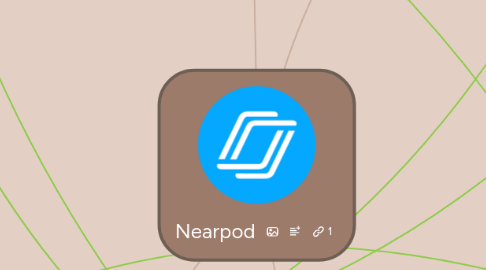# NearpodSusan Ramirez
Get Started. It's FreeNearpod## 1. Learning Goals/Objectives

### 1.1. Create

1.1.1. Research

1.1.2. Present

1.1.3. Write

1.1.3.1. Draw it!

1.1.3.1.1. Students can draw out word problems. They can also draw out graphs, geometry concepts, tables, etc.

### 1.2. NCTM

1.2.1. NCTM Standards

1.2.1.1. Problem Solving

1.2.1.1.1. Solving problems in math and other fields

1.2.1.1.2. Change problem solving strategy when necessary

1.2.1.2. Reasoning and Proof

1.2.1.2.1. Creating own proofs for conjectures and concepts learned

1.2.1.2.2. Evaluating others proofs

1.2.1.2.3. Creating sound arguments to clearly explain ideas and theorems

1.2.1.3. Communication

1.2.1.3.1. Students will have the ability to concisely communicate their mathematical ideas with others

1.2.1.3.2. Precisely communicate mathematical ideas

1.2.1.3.3. Analyze other students mathematical ideas

1.2.1.4. Connections

1.2.1.4.1. Identify connections between mathematical concepts

1.2.1.4.2. Connect math to outside of the mathematics classroom

1.2.1.5. Representations

1.2.1.5.1. Find ways to represent mathematical ideas in order to better communicate

1.2.1.5.2. Model

1.2.2. Conceptual Understanding

1.2.3. Procedural Understanding

## 2. Learning Activities

### 2.1. Individual Work

2.1.1. Create and distribute quizzes via Nearpod

2.1.1.1. Nearpod allows teachers to observe all student activity. This feature can prevent cheating from occurring during quizzes and assessments.

2.1.2. Upload polls for students to vote on what topic(s) needs more understanding.

2.1.3. Interact with a student who is out sick as Nearpod allows students to join in discussion from anywhere.

2.1.4. Flip the classroom and have students create a assessments or quizzes for their peers.

2.1.5. When individually presenting, students can present their power point to every students iPad with Nearpod.

### 2.2. Group and Class Work

2.2.1. Use Nearpod to create a presentation on how Nearpod works.

2.2.2. Get to know students with ice breaker activities.

2.2.3. Include virtual reality (VR) to allow to students to visualize and gain better understanding.

2.2.3.1. Make word problems come to life by setting up the problem with virtual reality.

2.2.4. Nearpod allows for interactive activities. Presentations of lessons can be on multiple features at once.

2.2.5. Create interactive activities through the lesson presentation.

## 3. Teacher Roles

### 3.1. Informer

3.1.1. What is Nearpod?

3.1.2. Why use it in a classroom?

### 3.2. Monitor

3.2.1. During the presentation

3.2.1.1. Can eliminate students from being off task

3.2.1.2. Can control when students are able to contribute to the presentation

3.2.1.2.1. Drawing

3.2.1.2.2. Quizzes

### 3.3. Trainer

3.3.1. Demonstrate in class how to use it

### 3.4. Planner

3.4.1. Plan the lesson

3.4.2. Teach the lesson

### 3.5. Facilitator

3.5.1. Participation in the presentation

3.5.2. Class discussion

### 3.6. Assessor

3.6.1. Assess students quiz scores, polling answers, drawings, fill in the blank, and short answer questions

### 3.7. Diagnostician

3.7.1. During instruction

3.7.2. During the presentation

3.7.3. During discussion

3.8.1. Teaching

## 4. Learning Assessment

### 4.1. Formative

4.1.1. Survey on how students liked it

4.1.2. See if students are improving

4.1.3. Use the in-presentation polls and quizzes to asses students learning as you go along

4.1.4. Formal assessment of student learning

### 4.2. Summative

4.2.1. Distribute an end of chapter assessment via Nearpod to display questions.

4.2.1.1. Same option can be applied for semester finals.

4.2.2. Benchmark tests could be presented through Nearpod

### 4.3. Diagnostic

4.3.1. Quiz

4.3.1.1. When learning about integrals, students could take a quiz about derivatives, so that the teacher can see how comfortable their students are with a concept that leads to integration.

4.3.2. Poll

4.3.2.1. Students can rate how comfortable they feel with mathematical concepts, so that the teacher can have a feel for the class environment. In a geometry classroom, students may be told to rate how well they believe they know the area of 2-d and 3-d shapes.

4.3.3. Collaborate

4.3.3.1. When beginning to talk about any new mathematical theorem or concept, students can discuss what they already know with this tool. They might write down anything they know or have learned in previous classes, so that other students may be reminded of things they have forgotten they have learned.

4.3.4. Open Ended Question

4.3.4.1. Students may be asked to explain the difference between mode, range, mean/average, etc. in a statistics class.